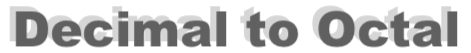How to convert 18 to octalHere we will show you step-by-step how convert the decimal number 18 to octal.

First note that decimal numbers use 10 digits (0, 1, 2, 3, 4, 5, 6, 7, 8, and 9) and octal numbers use only 8 digits (0, 1, 2, 3, 4, 5, 6, and 7).

As we explain the steps to converting 18 to octal, it is important to know the name of the parts of a division problem. In a problem like A divided by B equals C, A is the dividend, B is the divisor and C is the Quotient.

The Quotient has two parts. The Whole part and the Fractional part. The Fractional part is also known as the Remainder.

Step 1) Divide 18 by 8 to get the Quotient. Keep the Whole part for the next step and set the Remainder aside.

Step 2) Divide the Whole part of the Quotient from step 1 by 8. Again keep the Whole part and set the Remainder aside.

Step 3) Repeat Step 2 above until the Whole part is 0.

Step 4) Write down the Remainders in reverse order to get the answer to 18 as an octal.

Here we will show our work so you can follow along:

18 / 8 = 2 with 2 remainder
2 / 8 = 0 with 2 remainder

Then, when we put the remainders together in reverse order, we get the answer. The decimal number 18 converted to octal is therefore:

22

So what we did on the page was to Convert A10 to B8, where A is the decimal number 18 and B is the octal number 22. Which means that you can display decimal number 18 to octal in mathematical terms as follows:

1810 = 228

Decimal to Octal Converter
Need another decimal number as an octal number? How to convert 18 to octal is not all we know. Convert another decimal to octal below:

How to convert 19 to octal
Here is our next decimal to octal tutorial on our list.